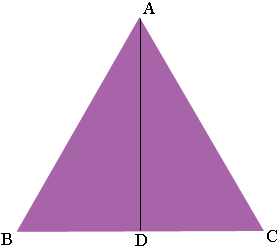SEARCH HOMEMath Central Quandaries & QueriesQuestion from Cristal, a student: Hello :) How can i find the sides of an equilateral triangle when only given the height 10m?Hi Cristal,

Suppose the triangle is $ABC$ and the length of a side of the triangle is $a$ units. Let $D$ be the midpoint of $BC$ and draw a line from $A$ to $D.$You know that $|BD| = |DC| = \frac{a}{2}.$

What do you know about the triangle $ABD$ and $ADC?$

Write back if you need more help.
PennyMath Central is supported by the University of Regina and The Pacific Institute for the Mathematical Sciences.